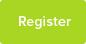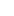# Tutor Hunt Questions

I have a data set consisting of three variables. I am a little uncertain as to whether or not to use a linear regression analysis or multiple regression.
The question I am investigating is `To what extent variable X or variable Y predict Variable K?`
Given that the question states OR rather than AND, I would assume linear regression? I can then compare the outcomes for both variables and say which variable is a better predictor?

Any help would be greatly appreciated!
2 years ago

Psychology Question asked by Erin

Hi Erin, you are correct. There are two separate questions here and then a comparison required. So answer to what extent doe X predict K and then to what extent does Y predict K and then compare. Hope that helps. Ben
22/11/2016 15:19:35 | comment by Ben

Please enter your response to the question below. The student will get a notification as soon your response has been approved by our moderation team.

You need to use multiple regression, though linear regression may not be the best fit. You should consider taking logs of your variables.

Not using multiple regression will not account for the correlation between x and y and lead to biased estimates. However, you may find that one of of x and y is not significant. You need to conduct a t-test to establish this.
Hi, My Name is Paulette and my answer is based on the content of your research question which was what statisticsal analysis would be appropriate to answer the question of the difference between how x versus y predicts k. You would a t-test on x then y with k as the dependent variable. So you wouldn’t use a multiple regression because you only have one dependent variable. You would use a linear regression since you have two independent variable. You would only do this see how both X and Y predicted K. To see which independent variable better predicts K, you would perform a Pearson correlation (r) -(xk) versus (yk) to see which is larger.
You can determine the separate correlations of x and y to k. Linear regression is a much more powerful test than the r correlation.
Because you are analysing two independent variables (x and y) then you would need to use linear regression. From my understanding you would use multiple regression when 2 correlated dependent variables are used (in this case you only have 1 dv which is k). Hope this helps, clare
Answered by Clare | 2 years ago